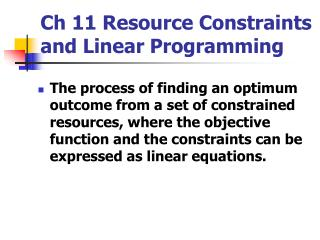DownloadDownload PresentationCh 11 Resource Constraints and Linear Programming

# Ch 11 Resource Constraints and Linear Programming

Télécharger la présentation## Ch 11 Resource Constraints and Linear Programming

- - - - - - - - - - - - - - - - - - - - - - - - - - - E N D - - - - - - - - - - - - - - - - - - - - - - - - - - -
##### Presentation Transcript

1. Ch 11 Resource Constraints and Linear Programming • The process of finding an optimum outcome from a set of constrained resources, where the objective function and the constraints can be expressed as linear equations.

2. Drawing the Linear Model

3. Adding the Linear Constraints Feasible Region

4. Adding the Iso-Contribution Line • The iso-contribution line is a ‘slope’ which represents the objective function. It is drawn as a generic line, then ‘floated’ to an optimum location within the feasible region.

5. Finding the Optimum Point • Float the iso-contribution line to an optimum position. Optimum point.

6. Algebraic Solution to an Example LP Problem • Define the objective function: Z = 0.75 X + 1.82 Y • Set up the resource constraints : • 32X + 59 Y <= 4312 • 200X + 15Y <= 1819 • Set up any other limit constraints; e.g; X >= 0 Y >= 0 X <= 19

7. Solving the Algebraic Problem 1 • In this simple case, the set of algebraic equations can be easily solved by substitution. • In a more complex case, the Simplex method can be manually applied. • As the Simplex method is tedious, and prone to error, the solution is best found with computer software such as Excel Solver. • The standard Excel spreadsheet needs to be specially adapted to run Solver.

8. Solving the Algebraic Problem 2 • Additions to the standard spreadsheet are: • An ‘Activity Level’ row for output levels. • A ‘Resource Supply’ column for level of supply of constrained resources. • A ‘Resource Use’ column for amount of each constrained resource used, and final objective function value. • A ‘Sign’ column for the inequality signs:- ( for information only; not for “Solver” solution.)